# Selina Solutions Concise Mathematics Class 6 Chapter 8: HCF And LCM

Selina Solutions are useful resources for students to follow during examinations. Frequent practice of Selina Solutions helps students to gain expertise in Mathematics. The answers are well structured, as per the students’ understanding capacity in solving the questions with ease. Students who find Mathematics difficult are advised to practice Selina Solutions effectively to boost confidence and face the exams without any fear

HCF is the greatest factor common to any two or more natural numbers and LCM is the lowest common multiple of any two or more natural numbers. Step by step solutions helps students in clearing their doubts, which appear while solving textbook questions. Our subject experts have prepared the solutions with the aim to help students in scoring good marks in the final exams. Students can download the PDF of Selina Solutions Concise Mathematics Class 6 Chapter 8 HCF and LCM, from the available links below

## Selina Solutions Concise Mathematics Class 6 Chapter 8: HCF And LCM Download PDF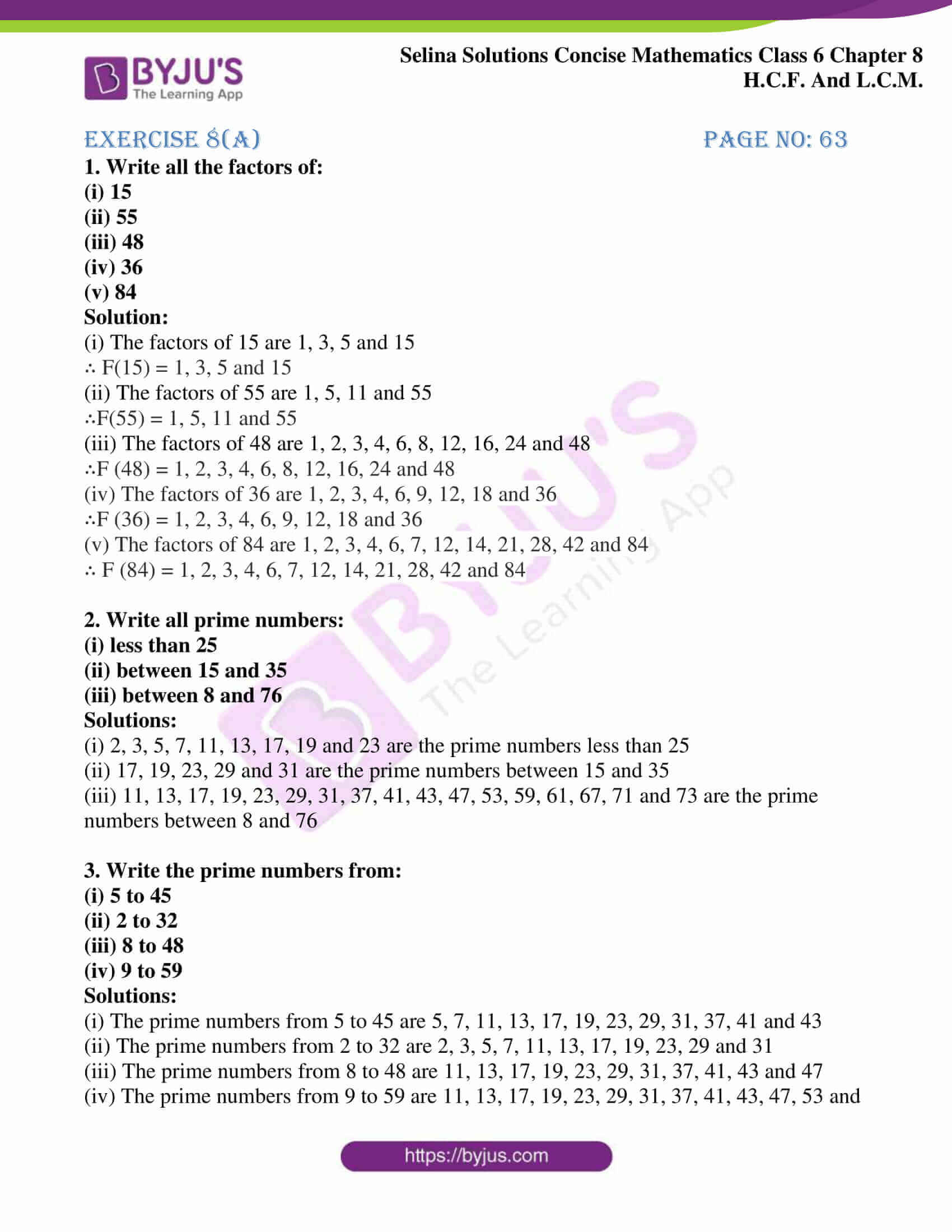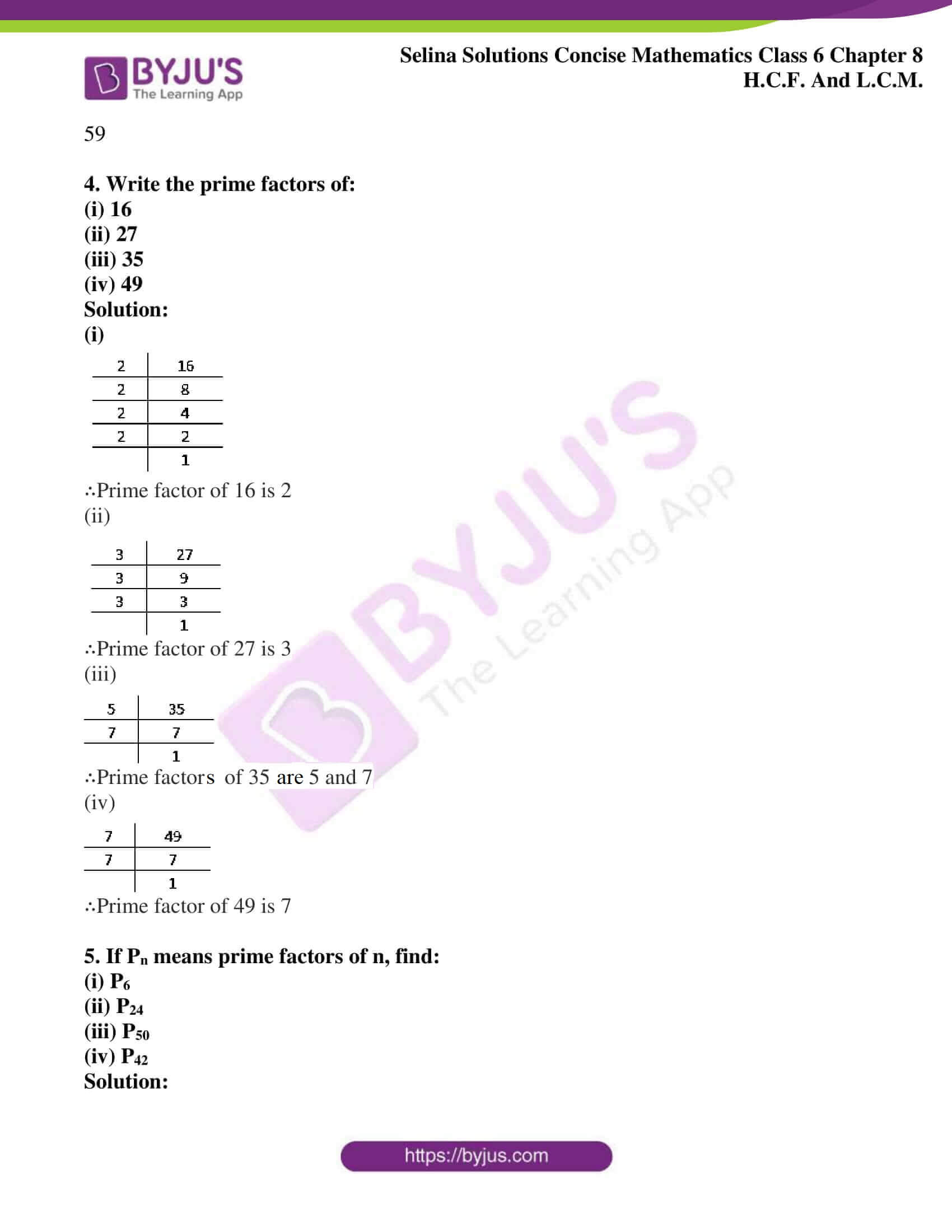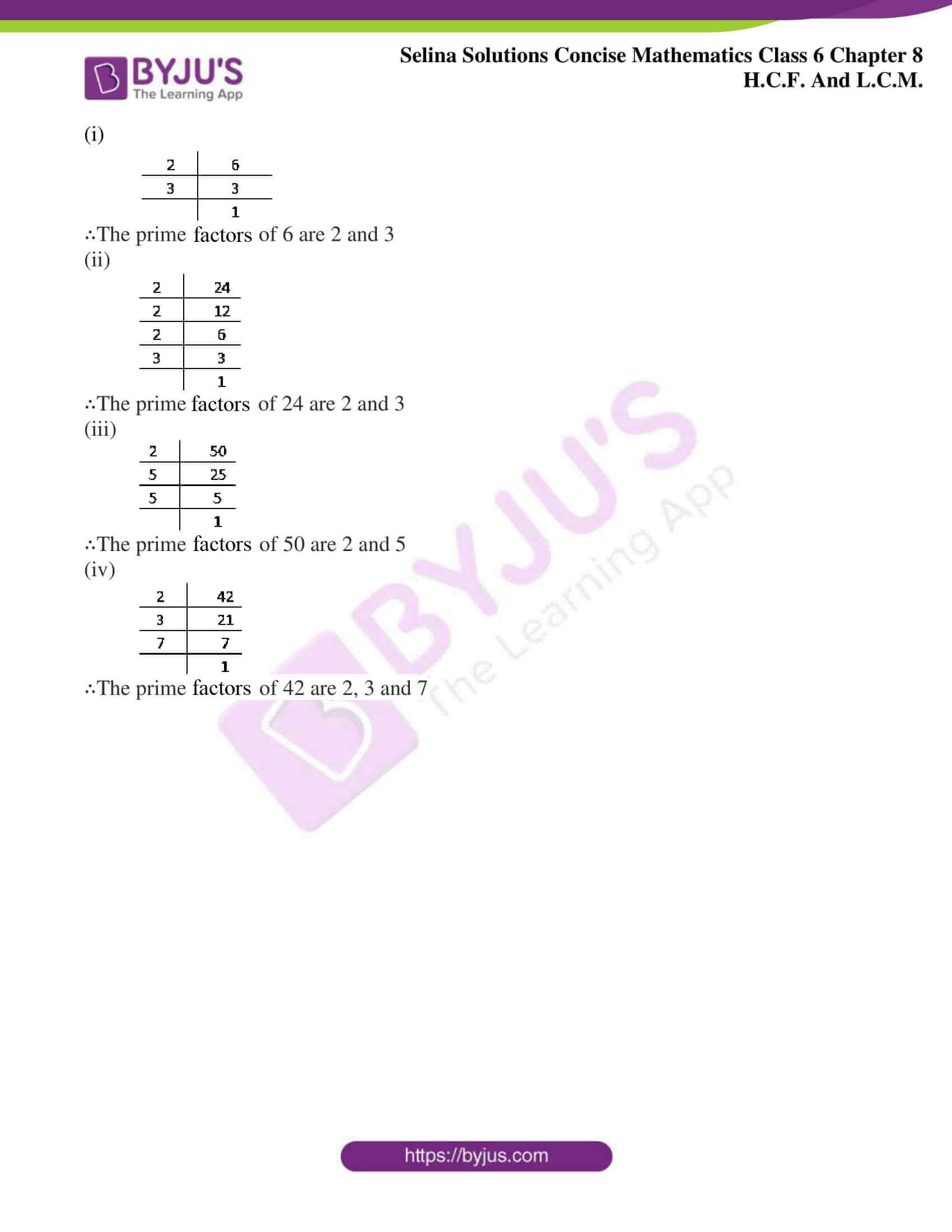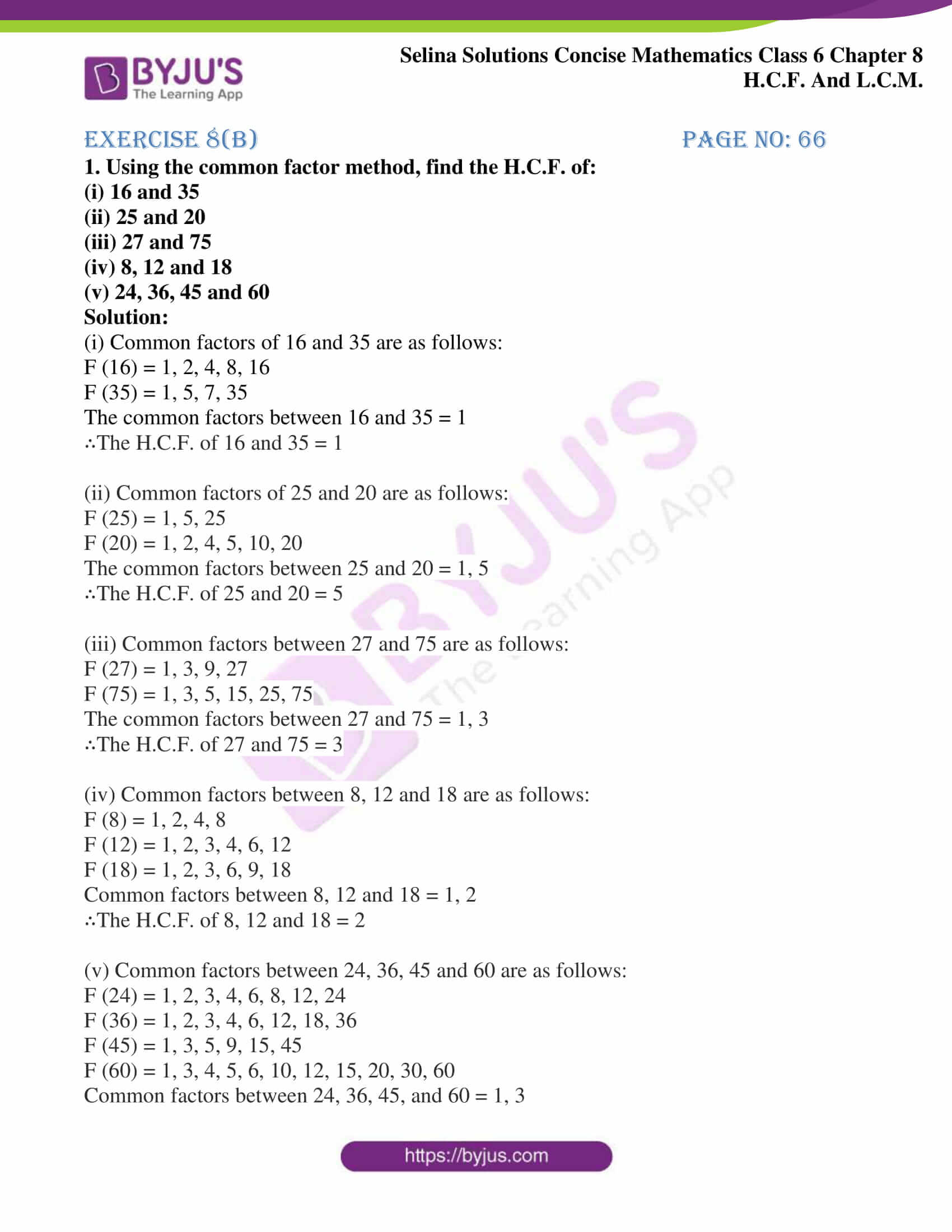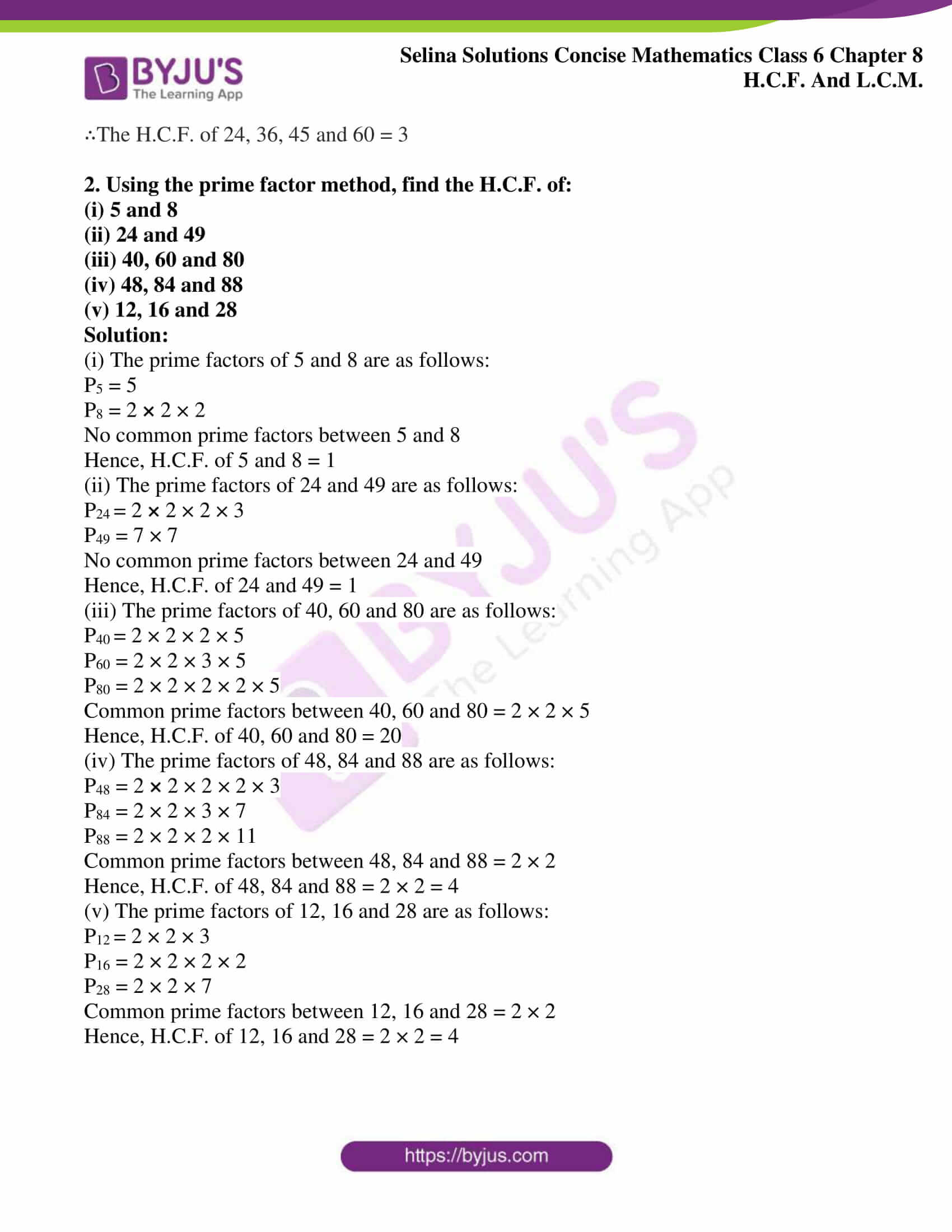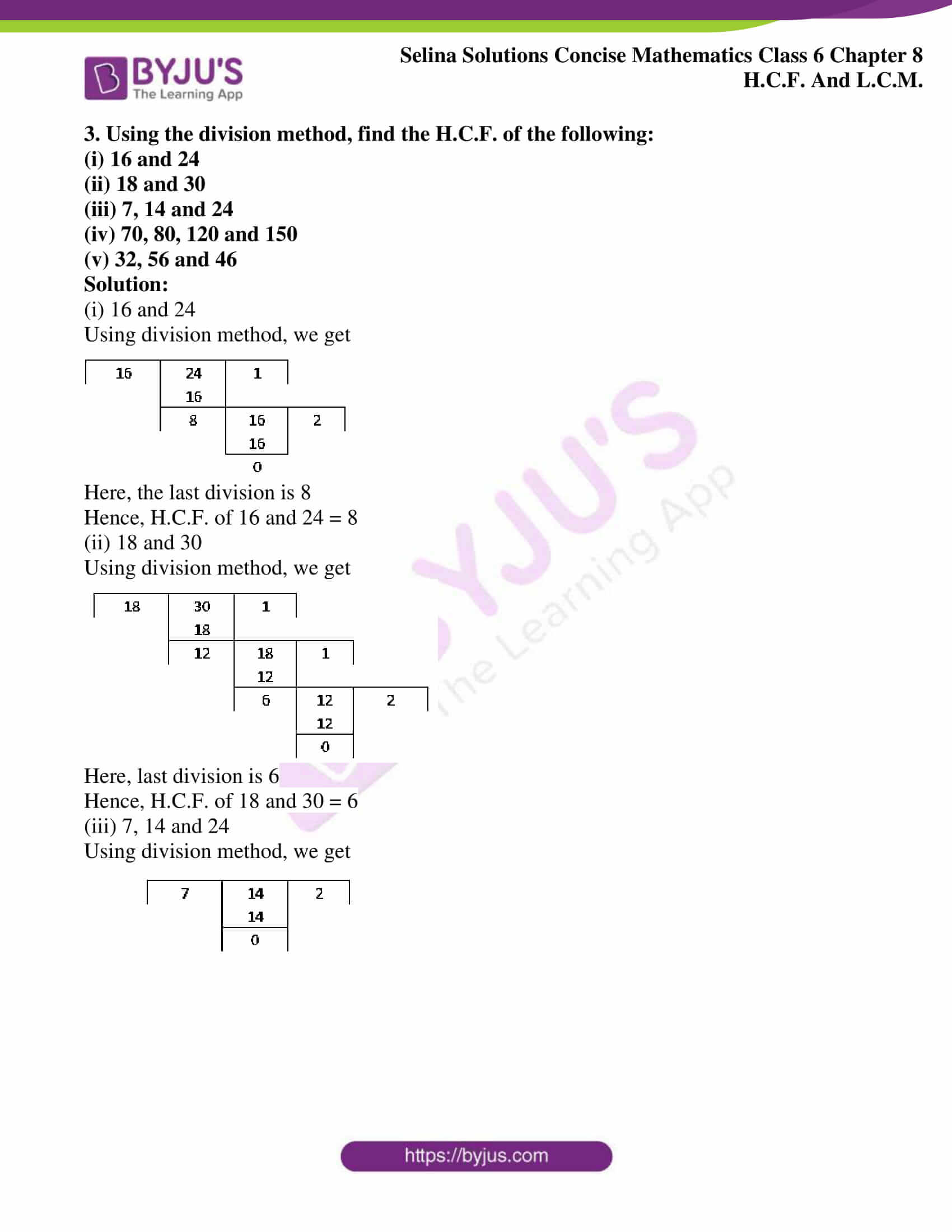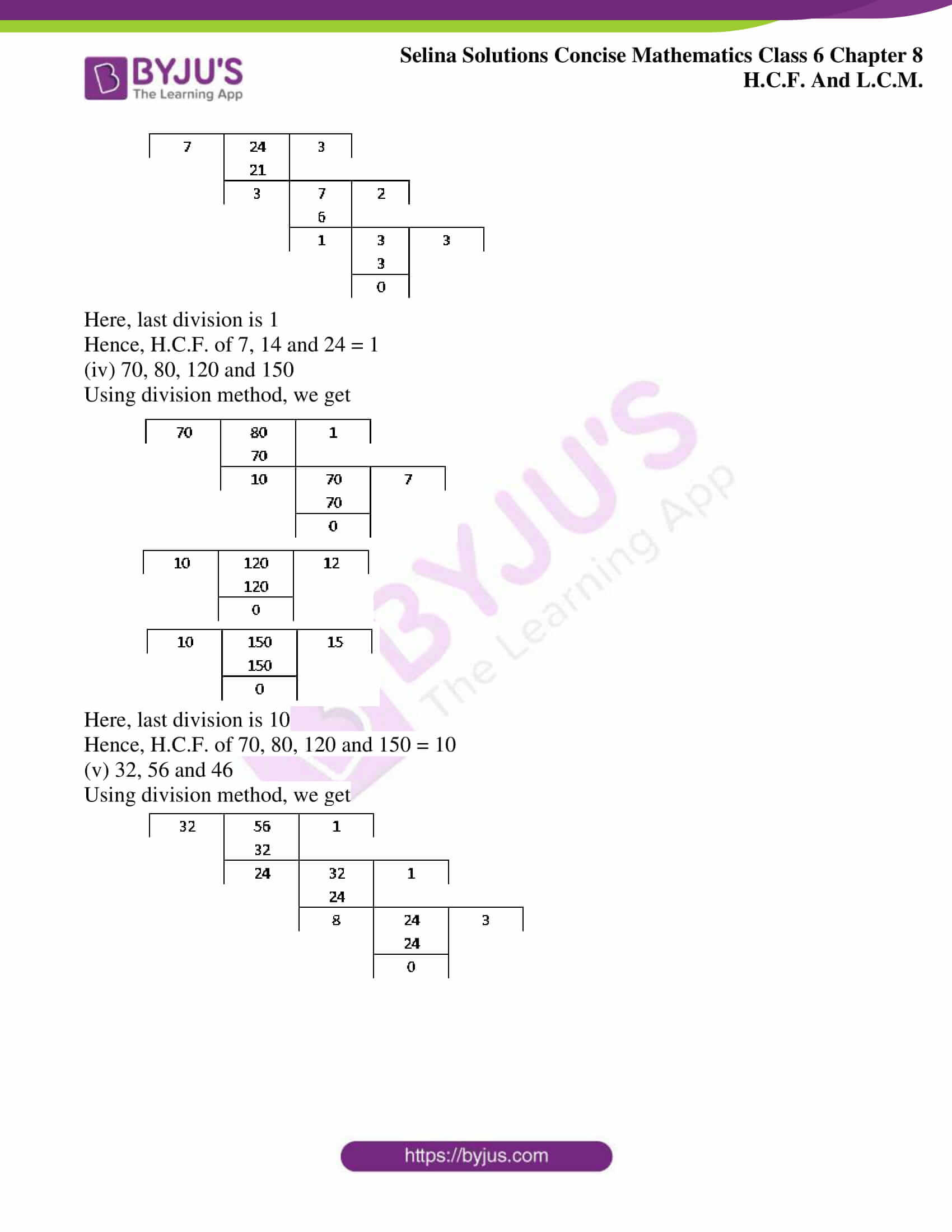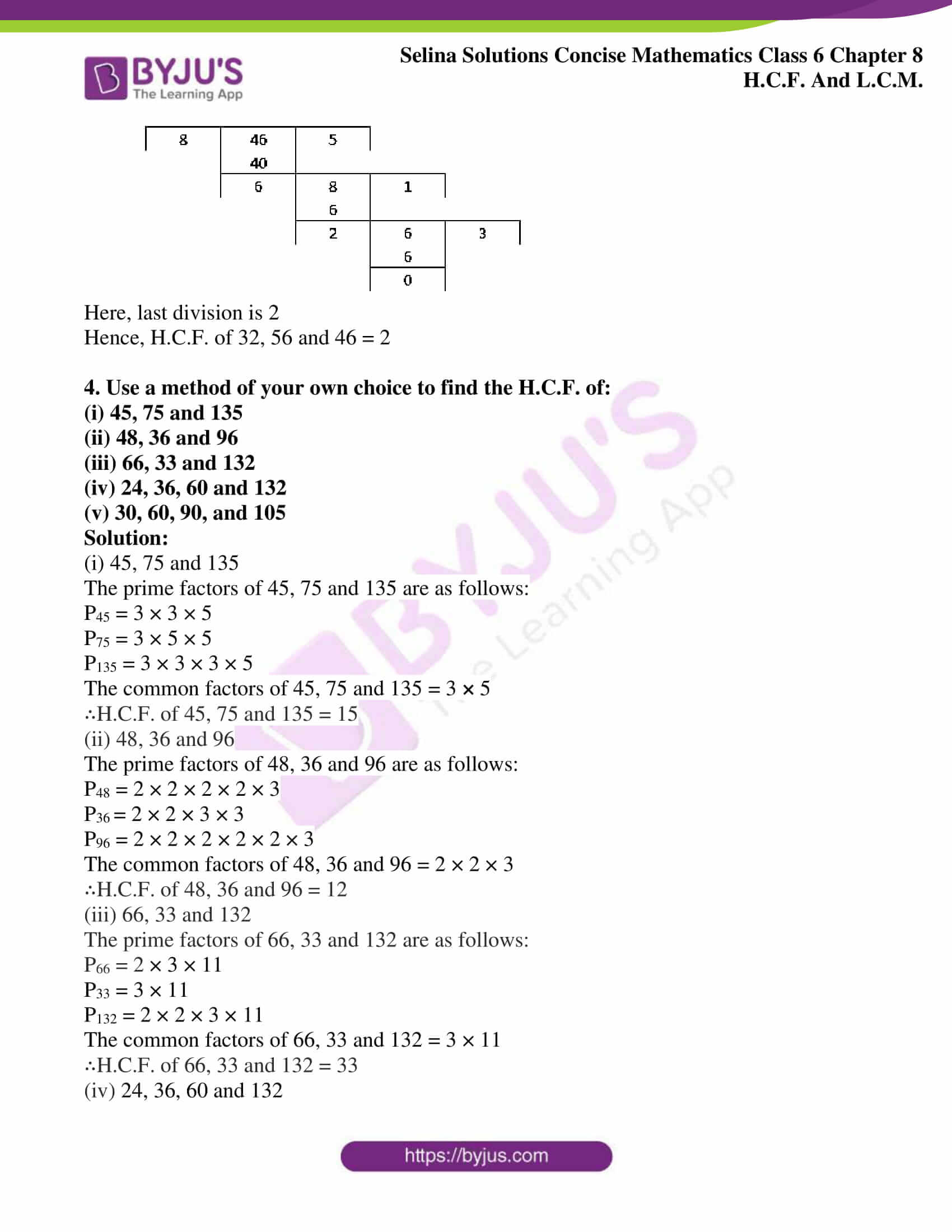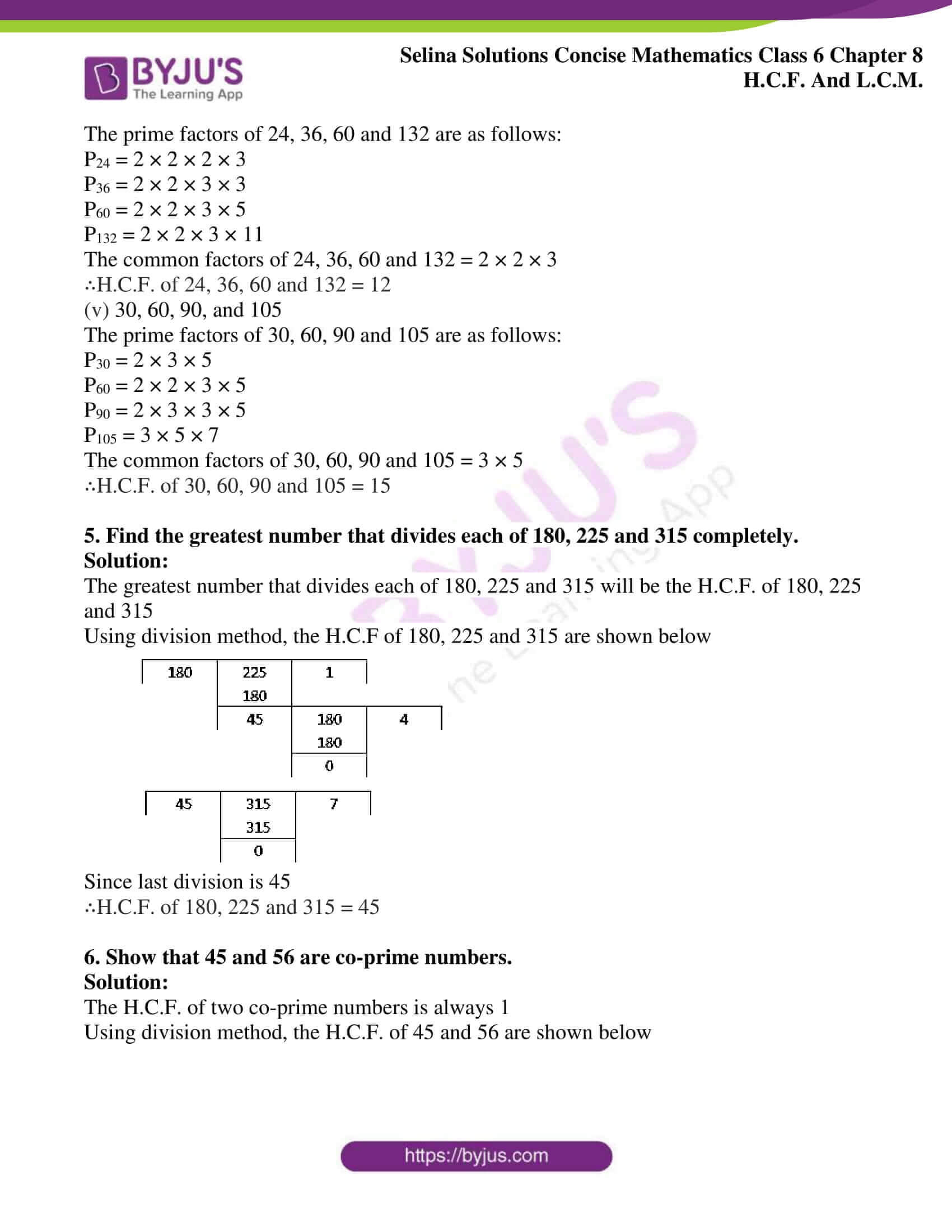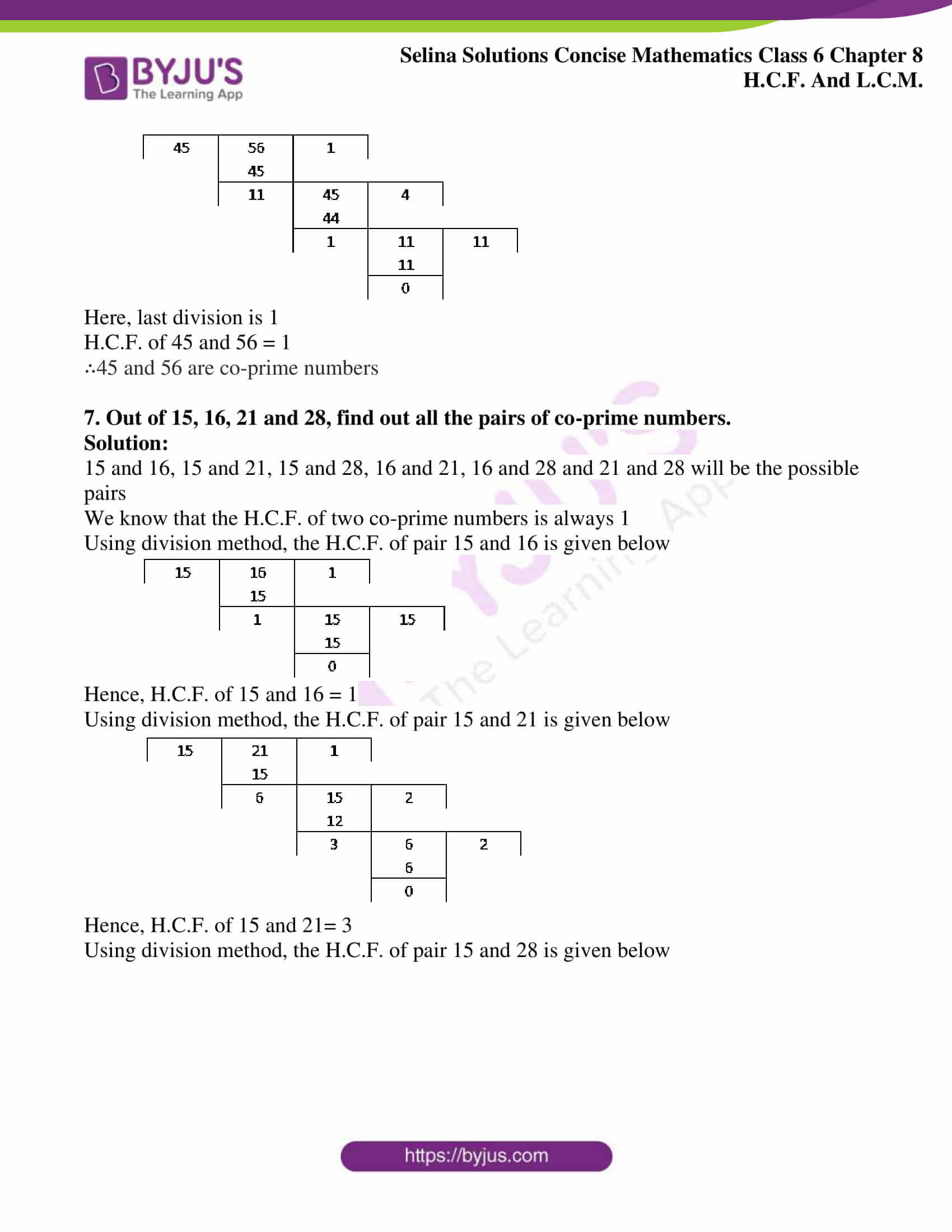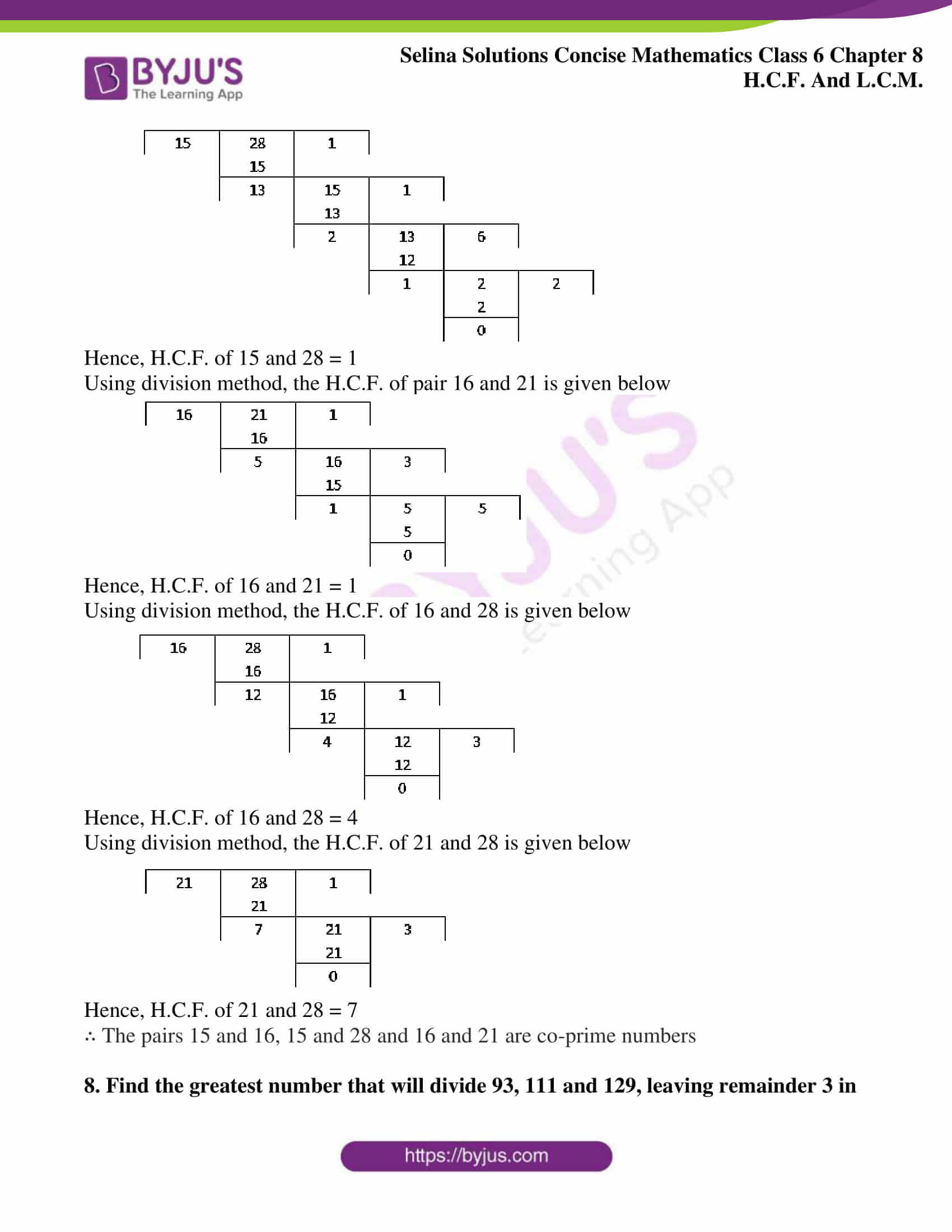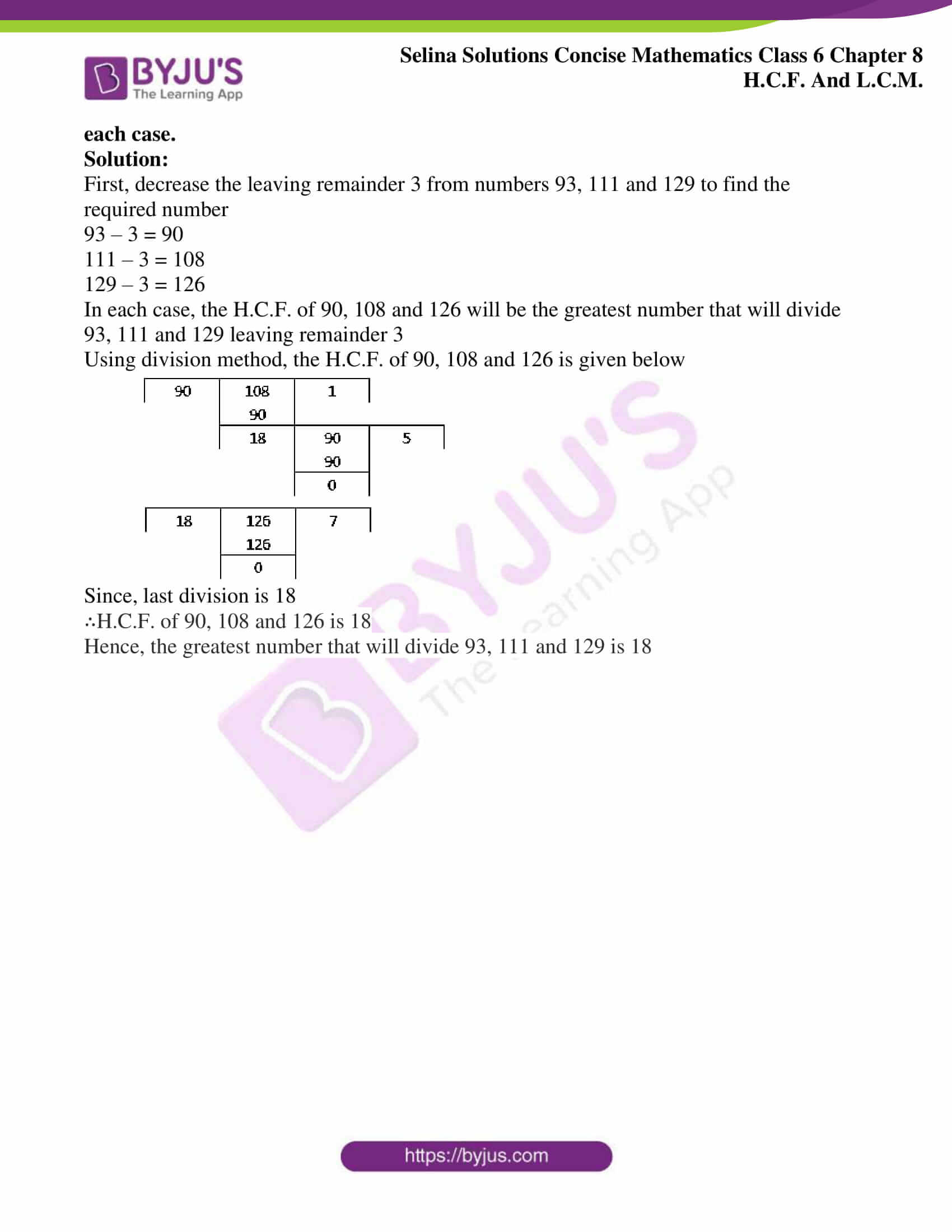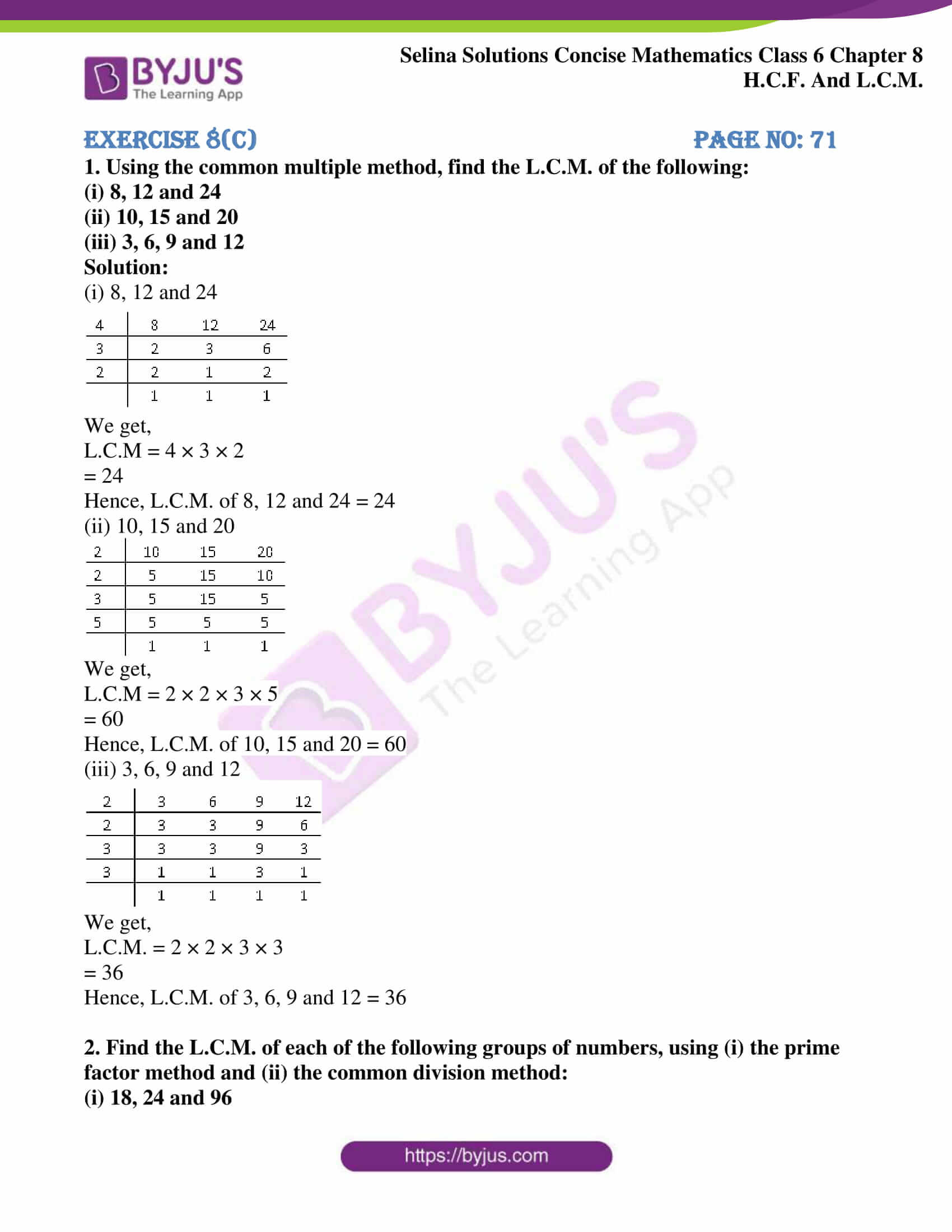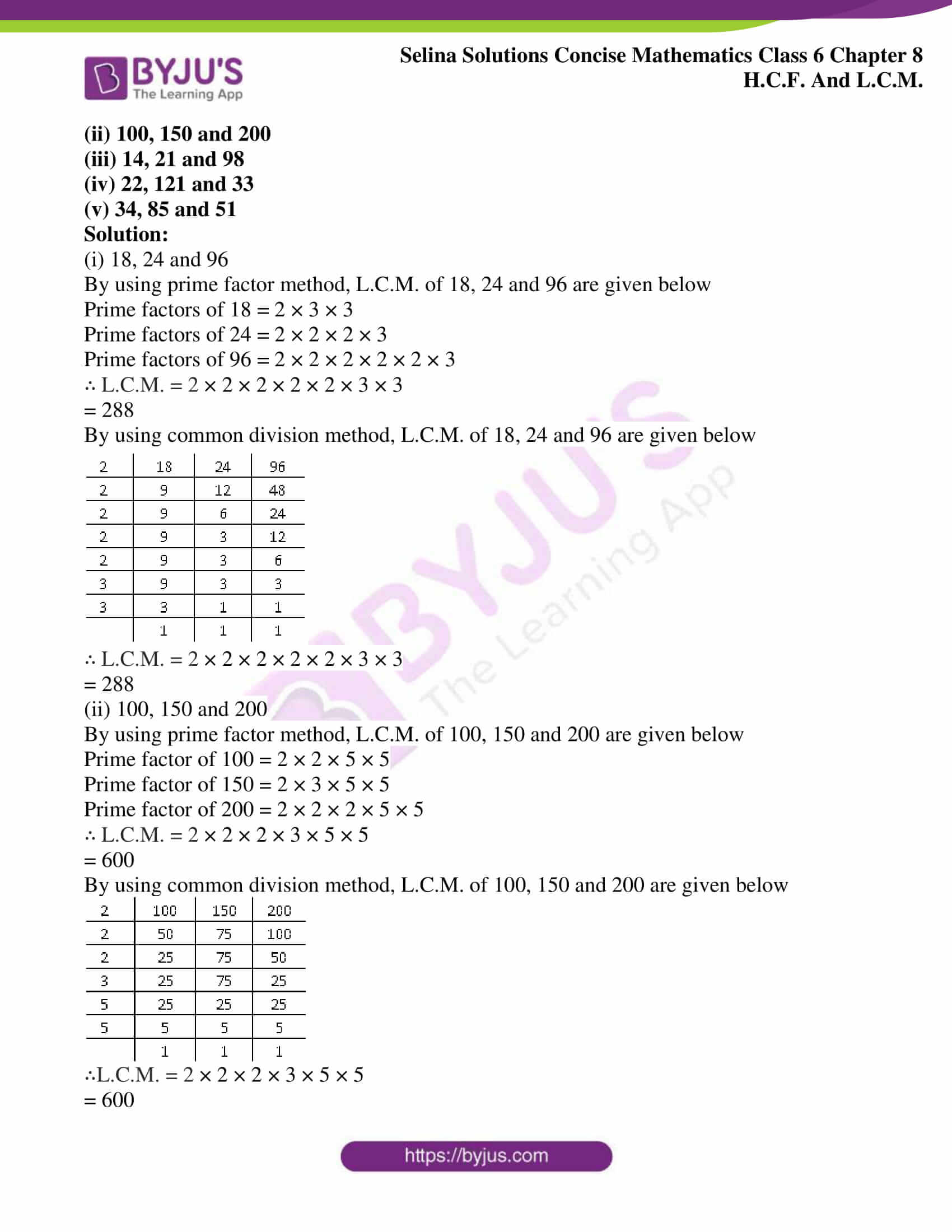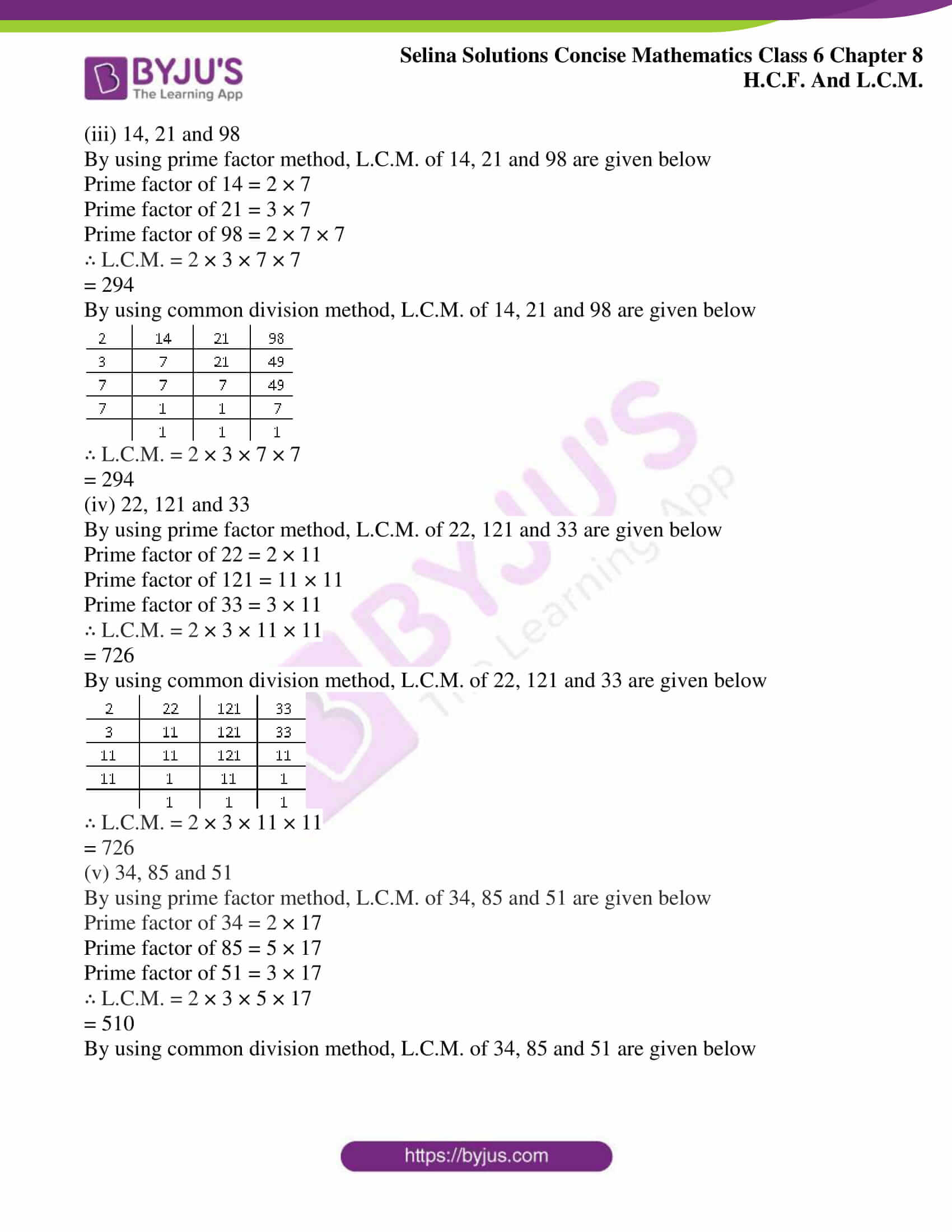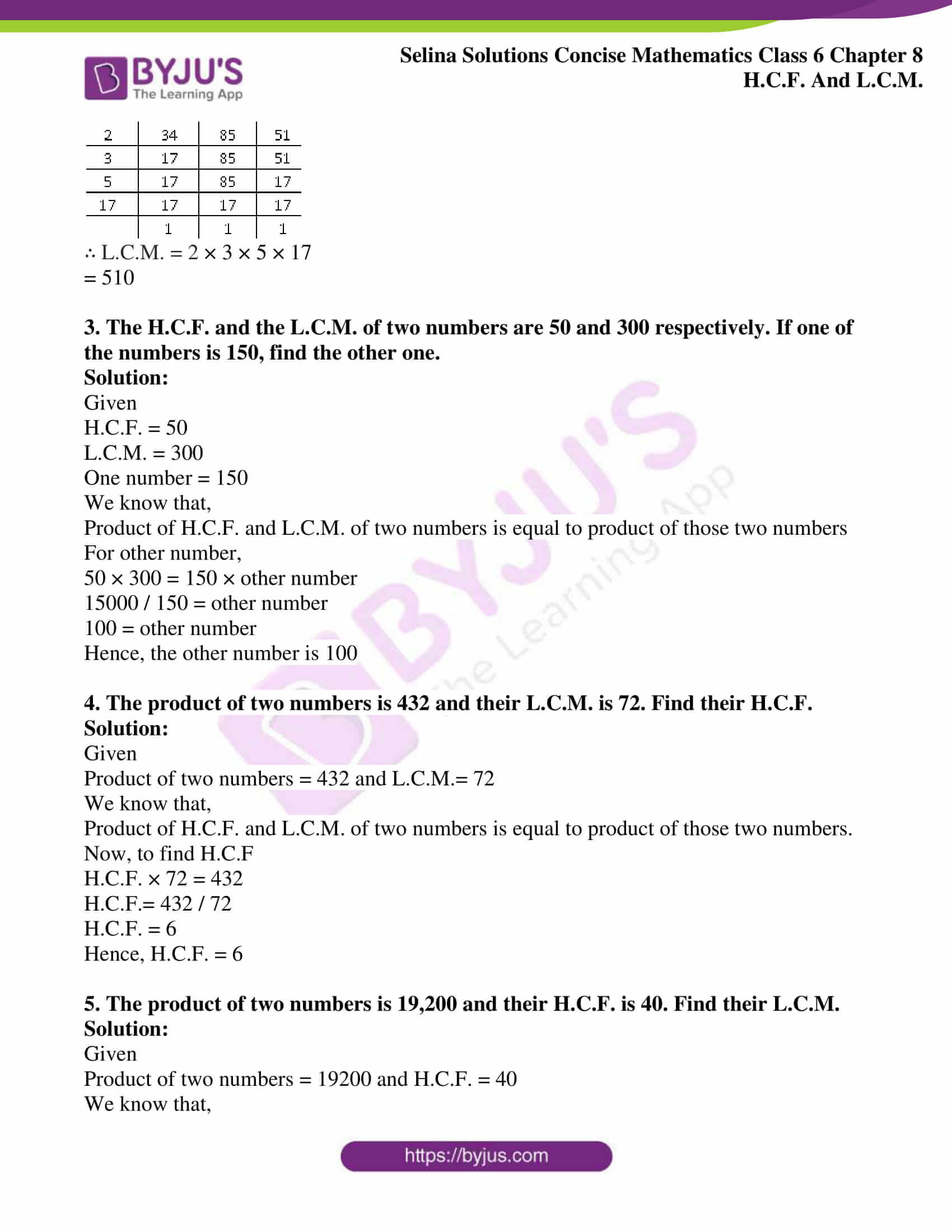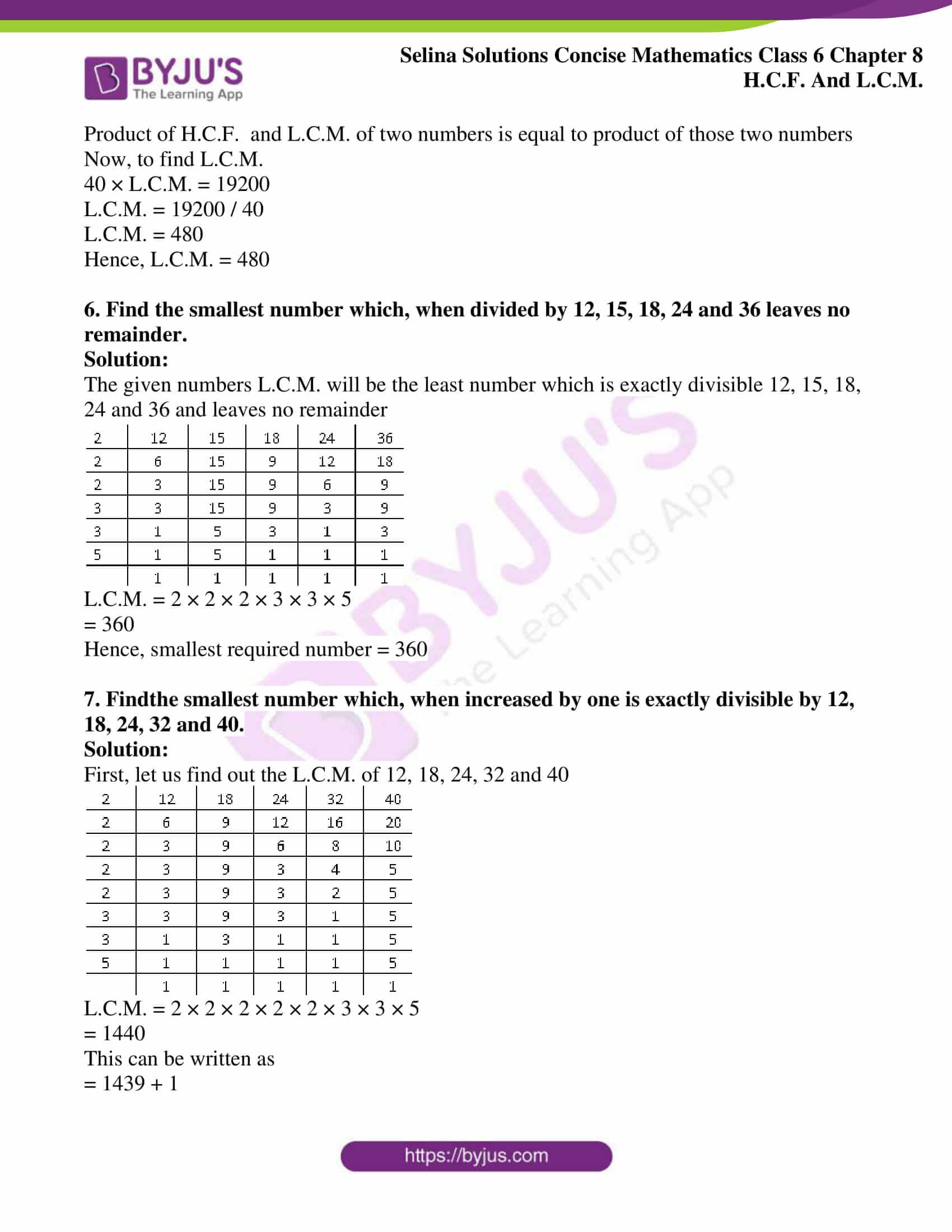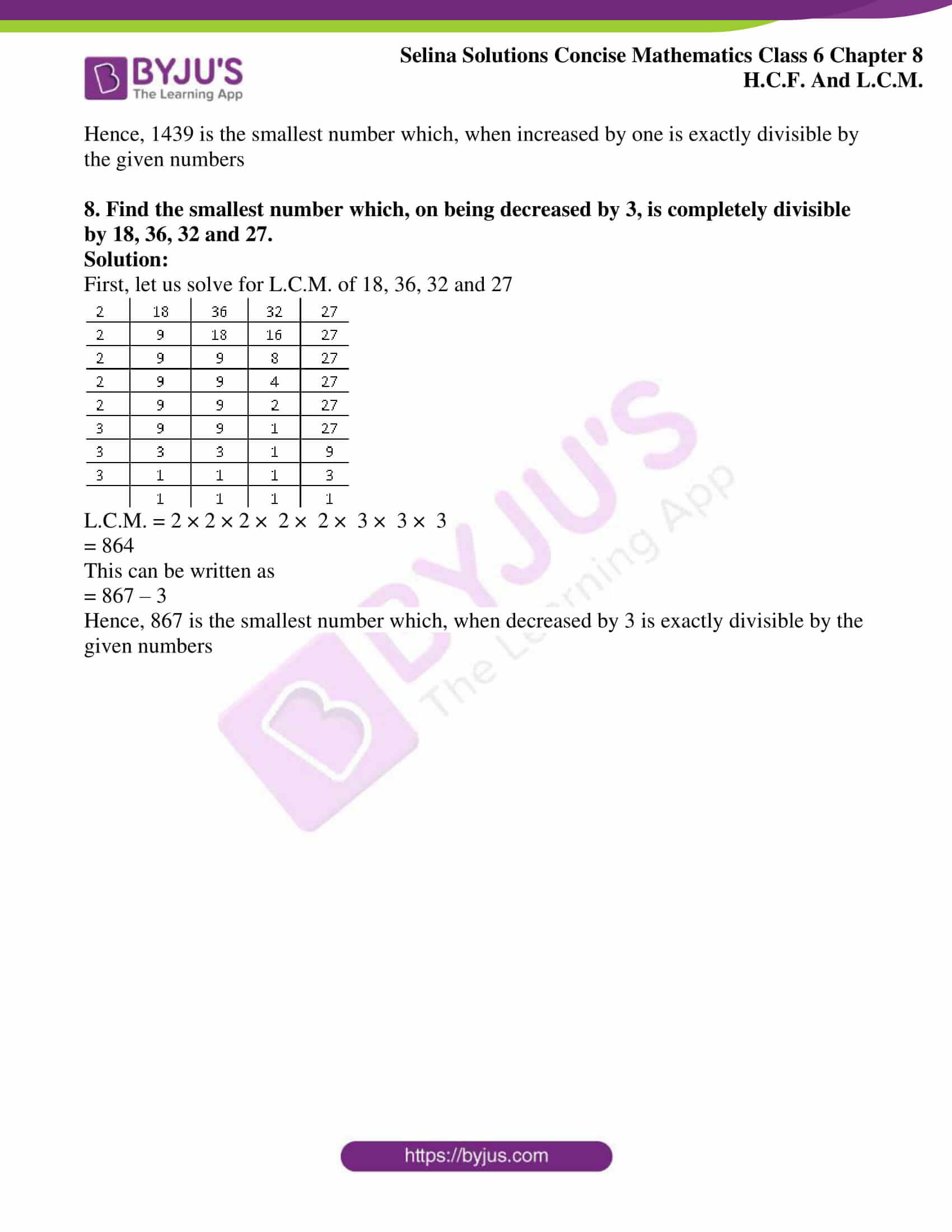### Exercises of Selina Solutions Concise Mathematics Class 6 Chapter 8: HCF And LCM

Exercise 8(A) Solutions

Exercise 8(B) Solutions

Exercise 8(C) Solutions

## Access Selina Solutions Concise Mathematics Class 6 Chapter 8: HCF And LCM

#### Exercise 8(A) page No: 63

1. Write all the factors of:

(i) 15

(ii) 55
(iii) 48

(iv) 36

(v) 84

Solution:

(i) The factors of 15 are 1, 3, 5 and 15

∴ F(15) = 1, 3, 5 and 15

(ii) The factors of 55 are 1, 5, 11 and 55

∴F(55) = 1, 5, 11 and 55

(iii) The factors of 48 are 1, 2, 3, 4, 6, 8, 12, 16, 24 and 48

∴F (48) = 1, 2, 3, 4, 6, 8, 12, 16, 24 and 48

(iv) The factors of 36 are 1, 2, 3, 4, 6, 9, 12, 18 and 36

∴F (36) = 1, 2, 3, 4, 6, 9, 12, 18 and 36

(v) The factors of 84 are 1, 2, 3, 4, 6, 7, 12, 14, 21, 28, 42 and 84

∴ F (84) = 1, 2, 3, 4, 6, 7, 12, 14, 21, 28, 42 and 84

2. Write all prime numbers:

(i) less than 25

(ii) between 15 and 35

(iii) between 8 and 76

Solutions:

(i) 2, 3, 5, 7, 11, 13, 17, 19 and 23 are the prime numbers less than 25

(ii) 17, 19, 23, 29 and 31 are the prime numbers between 15 and 35

(iii) 11, 13, 17, 19, 23, 29, 31, 37, 41, 43, 47, 53, 59, 61, 67, 71 and 73 are the prime numbers between 8 and 76

3. Write the prime numbers from:

(i) 5 to 45

(ii) 2 to 32

(iii) 8 to 48

(iv) 9 to 59

Solutions:

(i) The prime numbers from 5 to 45 are 5, 7, 11, 13, 17, 19, 23, 29, 31, 37, 41 and 43

(ii) The prime numbers from 2 to 32 are 2, 3, 5, 7, 11, 13, 17, 19, 23, 29 and 31

(iii) The prime numbers from 8 to 48 are 11, 13, 17, 19, 23, 29, 31, 37, 41, 43 and 47

(iv) The prime numbers from 9 to 59 are 11, 13, 17, 19, 23, 29, 31, 37, 41, 43, 47, 53 and 59

4. Write the prime factors of:

(i) 16

(ii) 27

(iii) 35

(iv) 49

Solution:

(i)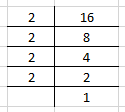∴Prime factor of 16 is 2

(ii)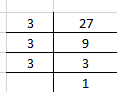∴Prime factor of 27 is 3

(iii)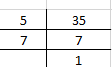∴Prime factors of 35 are 5 and 7

(iv)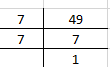∴Prime factor of 49 is 7

5. If Pn means prime factors of n, find:

(i) P6

(ii) P24

(iii) P50

(iv) P42

Solution:

(i)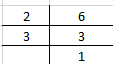∴The prime factors of 6 are 2 and 3

(ii)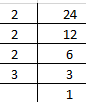∴The prime factors of 24 are 2 and 3

(iii)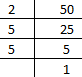∴The prime factors of 50 are 2 and 5

(iv)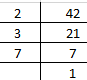∴The prime factors of 42 are 2, 3 and 7

#### Exercise 8(B) Page no: 66

1. Using the common factor method, find the H.C.F. of:

(i) 16 and 35

(ii) 25 and 20

(iii) 27 and 75

(iv) 8, 12 and 18

(v) 24, 36, 45 and 60

Solution:

(i) Common factors of 16 and 35 are as follows:

F (16) = 1, 2, 4, 8, 16

F (35) = 1, 5, 7, 35

The common factors between 16 and 35 = 1

∴The H.C.F. of 16 and 35 = 1

(ii) Common factors of 25 and 20 are as follows:

F (25) = 1, 5, 25

F (20) = 1, 2, 4, 5, 10, 20

The common factors between 25 and 20 = 1, 5

∴The H.C.F. of 25 and 20 = 5

(iii) Common factors between 27 and 75 are as follows:

F (27) = 1, 3, 9, 27

F (75) = 1, 3, 5, 15, 25, 75

The common factors between 27 and 75 = 1, 3

∴The H.C.F. of 27 and 75 = 3

(iv) Common factors between 8, 12 and 18 are as follows:

F (8) = 1, 2, 4, 8

F (12) = 1, 2, 3, 4, 6, 12

F (18) = 1, 2, 3, 6, 9, 18

Common factors between 8, 12 and 18 = 1, 2

∴The H.C.F. of 8, 12 and 18 = 2

(v) Common factors between 24, 36, 45 and 60 are as follows:

F (24) = 1, 2, 3, 4, 6, 8, 12, 24

F (36) = 1, 2, 3, 4, 6, 12, 18, 36

F (45) = 1, 3, 5, 9, 15, 45

F (60) = 1, 3, 4, 5, 6, 10, 12, 15, 20, 30, 60

Common factors between 24, 36, 45, and 60 = 1, 3

∴The H.C.F. of 24, 36, 45 and 60 = 3

2. Using the prime factor method, find the H.C.F. of:

(i) 5 and 8

(ii) 24 and 49

(iii) 40, 60 and 80

(iv) 48, 84 and 88

(v) 12, 16 and 28

Solution:

(i) The prime factors of 5 and 8 are as follows:

P5 = 5

P8 = 2 × 2 × 2

No common prime factors between 5 and 8

Hence, H.C.F. of 5 and 8 = 1

(ii) The prime factors of 24 and 49 are as follows:

P24 = 2 × 2 × 2 × 3

P49 = 7 × 7

No common prime factors between 24 and 49

Hence, H.C.F. of 24 and 49 = 1

(iii) The prime factors of 40, 60 and 80 are as follows:

P40 = 2 × 2 × 2 × 5

P60 = 2 × 2 × 3 × 5

P80 = 2 × 2 × 2 × 2 × 5

Common prime factors between 40, 60 and 80 = 2 × 2 × 5

Hence, H.C.F. of 40, 60 and 80 = 20

(iv) The prime factors of 48, 84 and 88 are as follows:

P48 = 2 × 2 × 2 × 2 × 3

P84 = 2 × 2 × 3 × 7

P88 = 2 × 2 × 2 × 11

Common prime factors between 48, 84 and 88 = 2 × 2

Hence, H.C.F. of 48, 84 and 88 = 2 × 2 = 4

(v) The prime factors of 12, 16 and 28 are as follows:

P12 = 2 × 2 × 3

P16 = 2 × 2 × 2 × 2

P28 = 2 × 2 × 7

Common prime factors between 12, 16 and 28 = 2 × 2

Hence, H.C.F. of 12, 16 and 28 = 2 × 2 = 4

3. Using the division method, find the H.C.F. of the following:

(i) 16 and 24

(ii) 18 and 30

(iii) 7, 14 and 24

(iv) 70, 80, 120 and 150

(v) 32, 56 and 46

Solution:

(i) 16 and 24

Using division method, we get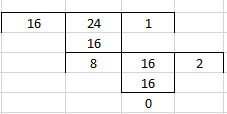Here, the last division is 8

Hence, H.C.F. of 16 and 24 = 8

(ii) 18 and 30

Using division method, we get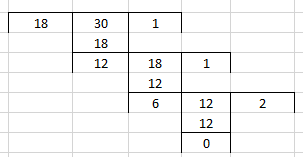Here, last division is 6

Hence, H.C.F. of 18 and 30 = 6

(iii) 7, 14 and 24

Using division method, we get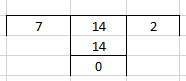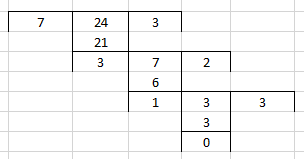Here, last division is 1

Hence, H.C.F. of 7, 14 and 24 = 1

(iv) 70, 80, 120 and 150

Using division method, we get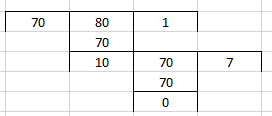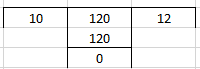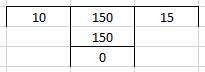Here, last division is 10

Hence, H.C.F. of 70, 80, 120 and 150 = 10

(v) 32, 56 and 46

Using division method, we get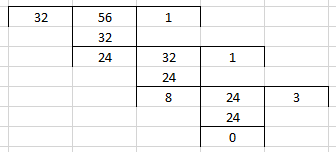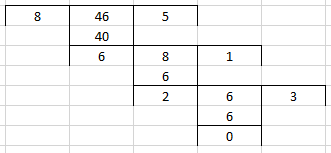Here, last division is 2

Hence, H.C.F. of 32, 56 and 46 = 2

4. Use a method of your own choice to find the H.C.F. of:

(i) 45, 75 and 135

(ii) 48, 36 and 96

(iii) 66, 33 and 132

(iv) 24, 36, 60 and 132

(v) 30, 60, 90, and 105

Solution:

(i) 45, 75 and 135

The prime factors of 45, 75 and 135 are as follows:

P45 = 3 × 3 × 5

P75 = 3 × 5 × 5

P135 = 3 × 3 × 3 × 5

The common factors of 45, 75 and 135 = 3 × 5

∴H.C.F. of 45, 75 and 135 = 15

(ii) 48, 36 and 96

The prime factors of 48, 36 and 96 are as follows:

P48 = 2 × 2 × 2 × 2 × 3

P36 = 2 × 2 × 3 × 3

P96 = 2 × 2 × 2 × 2 × 2 × 3

The common factors of 48, 36 and 96 = 2 × 2 × 3

∴H.C.F. of 48, 36 and 96 = 12

(iii) 66, 33 and 132

The prime factors of 66, 33 and 132 are as follows:

P66 = 2 × 3 × 11

P33 = 3 × 11

P132 = 2 × 2 × 3 × 11

The common factors of 66, 33 and 132 = 3 × 11

∴H.C.F. of 66, 33 and 132 = 33

(iv) 24, 36, 60 and 132

The prime factors of 24, 36, 60 and 132 are as follows:

P24 = 2 × 2 × 2 × 3

P36 = 2 × 2 × 3 × 3

P60 = 2 × 2 × 3 × 5

P132 = 2 × 2 × 3 × 11

The common factors of 24, 36, 60 and 132 = 2 × 2 × 3

∴H.C.F. of 24, 36, 60 and 132 = 12

(v) 30, 60, 90, and 105

The prime factors of 30, 60, 90 and 105 are as follows:

P30 = 2 × 3 × 5

P60 = 2 × 2 × 3 × 5

P90 = 2 × 3 × 3 × 5

P105 = 3 × 5 × 7

The common factors of 30, 60, 90 and 105 = 3 × 5

∴H.C.F. of 30, 60, 90 and 105 = 15

5. Find the greatest number that divides each of 180, 225 and 315 completely.

Solution:

The greatest number that divides each of 180, 225 and 315 will be the H.C.F. of 180, 225 and 315

Using division method, the H.C.F of 180, 225 and 315 are shown below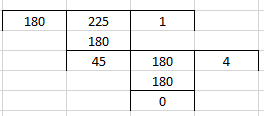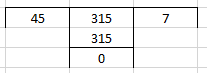Since last division is 45

∴H.C.F. of 180, 225 and 315 = 45

6. Show that 45 and 56 are co-prime numbers.

Solution:

The H.C.F. of two co-prime numbers is always 1

Using division method, the H.C.F. of 45 and 56 are shown below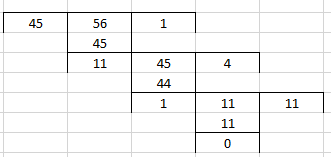Here, last division is 1

H.C.F. of 45 and 56 = 1

∴45 and 56 are co-prime numbers

7. Out of 15, 16, 21 and 28, find out all the pairs of co-prime numbers.

Solution:

15 and 16, 15 and 21, 15 and 28, 16 and 21, 16 and 28 and 21 and 28 will be the possible pairs

We know that the H.C.F. of two co-prime numbers is always 1

Using division method, the H.C.F. of pair 15 and 16 is given below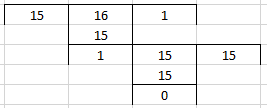Hence, H.C.F. of 15 and 16 = 1

Using division method, the H.C.F. of pair 15 and 21 is given below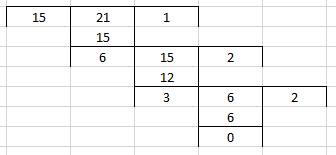Hence, H.C.F. of 15 and 21= 3

Using division method, the H.C.F. of pair 15 and 28 is given below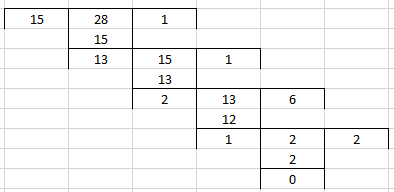Hence, H.C.F. of 15 and 28 = 1

Using division method, the H.C.F. of pair 16 and 21 is given below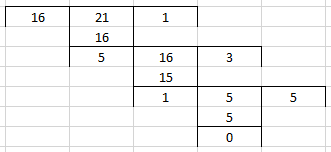Hence, H.C.F. of 16 and 21 = 1

Using division method, the H.C.F. of 16 and 28 is given below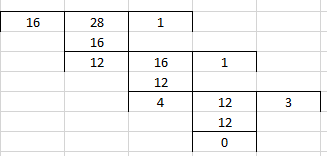Hence, H.C.F. of 16 and 28 = 4

Using division method, the H.C.F. of 21 and 28 is given below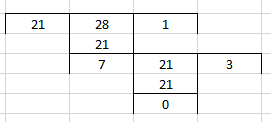Hence, H.C.F. of 21 and 28 = 7

∴ The pairs 15 and 16, 15 and 28 and 16 and 21 are co-prime numbers

8. Find the greatest number that will divide 93, 111 and 129, leaving remainder 3 in each case.

Solution:

First, decrease the leaving remainder 3 from numbers 93, 111 and 129 to find the required number

93 – 3 = 90

111 – 3 = 108

129 – 3 = 126

In each case, the H.C.F. of 90, 108 and 126 will be the greatest number that will divide 93, 111 and 129 leaving remainder 3

Using division method, the H.C.F. of 90, 108 and 126 is given below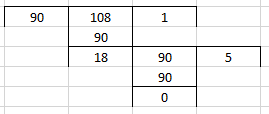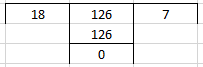Since, last division is 18

∴H.C.F. of 90, 108 and 126 is 18

Hence, the greatest number that will divide 93, 111 and 129 is 18

#### Exercise 8(C) page no: 71

1. Using the common multiple method, find the L.C.M. of the following:

(i) 8, 12 and 24

(ii) 10, 15 and 20

(iii) 3, 6, 9 and 12

Solution:

(i) 8, 12 and 24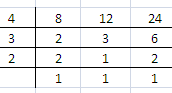We get,

L.C.M = 4 × 3 × 2

= 24

Hence, L.C.M. of 8, 12 and 24 = 24

(ii) 10, 15 and 20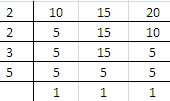We get,

L.C.M = 2 × 2 × 3 × 5

= 60

Hence, L.C.M. of 10, 15 and 20 = 60

(iii) 3, 6, 9 and 12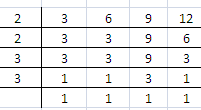We get,

L.C.M. = 2 × 2 × 3 × 3

= 36

Hence, L.C.M. of 3, 6, 9 and 12 = 36

2. Find the L.C.M. of each of the following groups of numbers, using (i) the prime factor method and (ii) the common division method:

(i) 18, 24 and 96

(ii) 100, 150 and 200

(iii) 14, 21 and 98

(iv) 22, 121 and 33

(v) 34, 85 and 51

Solution:

(i) 18, 24 and 96

By using prime factor method, L.C.M. of 18, 24 and 96 are given below

Prime factors of 18 = 2 × 3 × 3

Prime factors of 24 = 2 × 2 × 2 × 3

Prime factors of 96 = 2 × 2 × 2 × 2 × 2 × 3

∴ L.C.M. = 2 × 2 × 2 × 2 × 2 × 3 × 3

= 288

By using common division method, L.C.M. of 18, 24 and 96 are given below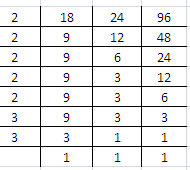∴ L.C.M. = 2 × 2 × 2 × 2 × 2 × 3 × 3

= 288

(ii) 100, 150 and 200

By using prime factor method, L.C.M. of 100, 150 and 200 are given below

Prime factor of 100 = 2 × 2 × 5 × 5

Prime factor of 150 = 2 × 3 × 5 × 5

Prime factor of 200 = 2 × 2 × 2 × 5 × 5

∴ L.C.M. = 2 × 2 × 2 × 3 × 5 × 5

= 600

By using common division method, L.C.M. of 100, 150 and 200 are given below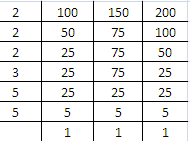∴L.C.M. = 2 × 2 × 2 × 3 × 5 × 5

= 600

(iii) 14, 21 and 98

By using prime factor method, L.C.M. of 14, 21 and 98 are given below

Prime factor of 14 = 2 × 7

Prime factor of 21 = 3 × 7

Prime factor of 98 = 2 × 7 × 7

∴ L.C.M. = 2 × 3 × 7 × 7

= 294

By using common division method, L.C.M. of 14, 21 and 98 are given below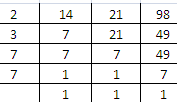∴ L.C.M. = 2 × 3 × 7 × 7

= 294

(iv) 22, 121 and 33

By using prime factor method, L.C.M. of 22, 121 and 33 are given below

Prime factor of 22 = 2 × 11

Prime factor of 121 = 11 × 11

Prime factor of 33 = 3 × 11

∴ L.C.M. = 2 × 3 × 11 × 11

= 726

By using common division method, L.C.M. of 22, 121 and 33 are given below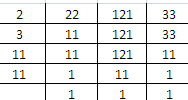∴ L.C.M. = 2 × 3 × 11 × 11

= 726

(v) 34, 85 and 51

By using prime factor method, L.C.M. of 34, 85 and 51 are given below

Prime factor of 34 = 2 × 17

Prime factor of 85 = 5 × 17

Prime factor of 51 = 3 × 17

∴ L.C.M. = 2 × 3 × 5 × 17

= 510

By using common division method, L.C.M. of 34, 85 and 51 are given below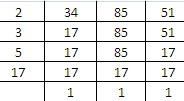∴ L.C.M. = 2 × 3 × 5 × 17

= 510

3. The H.C.F. and the L.C.M. of two numbers are 50 and 300 respectively. If one of the numbers is 150, find the other one.

Solution:

Given

H.C.F. = 50

L.C.M. = 300

One number = 150

We know that,

Product of H.C.F. and L.C.M. of two numbers is equal to product of those two numbers

For other number,

50 × 300 = 150 × other number

15000 / 150 = other number

100 = other number

Hence, the other number is 100

4. The product of two numbers is 432 and their L.C.M. is 72. Find their H.C.F.

Solution:

Given

Product of two numbers = 432 and L.C.M.= 72

We know that,

Product of H.C.F. and L.C.M. of two numbers is equal to product of those two numbers.

Now, to find H.C.F

H.C.F. × 72 = 432

H.C.F.= 432 / 72

H.C.F. = 6

Hence, H.C.F. = 6

5. The product of two numbers is 19,200 and their H.C.F. is 40. Find their L.C.M.

Solution:

Given

Product of two numbers = 19200 and H.C.F. = 40

We know that,

Product of H.C.F. and L.C.M. of two numbers is equal to product of those two numbers

Now, to find L.C.M.

40 × L.C.M. = 19200

L.C.M. = 19200 / 40

L.C.M. = 480

Hence, L.C.M. = 480

6. Find the smallest number which, when divided by 12, 15, 18, 24 and 36 leaves no remainder.

Solution:

The given numbers L.C.M. will be the least number which is exactly divisible 12, 15, 18, 24 and 36 and leaves no remainder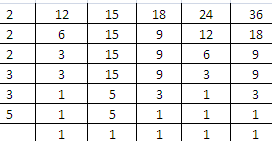L.C.M. = 2 × 2 × 2 × 3 × 3 × 5

= 360

Hence, smallest required number = 360

7. Find the smallest number which, when increased by one is exactly divisible by 12, 18, 24, 32 and 40.

Solution:

First, let us find out the L.C.M. of 12, 18, 24, 32 and 40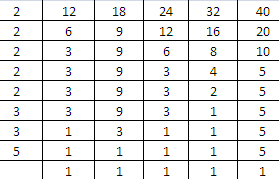L.C.M. = 2 × 2 × 2 × 2 × 2 × 3 × 3 × 5

= 1440

This can be written as

= 1439 + 1

Hence, 1439 is the smallest number which, when increased by one is exactly divisible by the given numbers

8. Find the smallest number which, on being decreased by 3, is completely divisible by 18, 36, 32 and 27.

Solution:

First, let us solve for L.C.M. of 18, 36, 32 and 27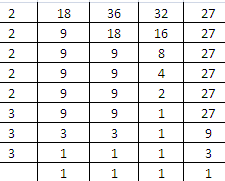L.C.M. = 2 × 2 × 2 × 2 × 2 × 3 × 3 × 3

= 864

This can be written as

= 867 – 3

Hence, 867 is the smallest number which, when decreased by 3 is exactly divisible by the given numbers